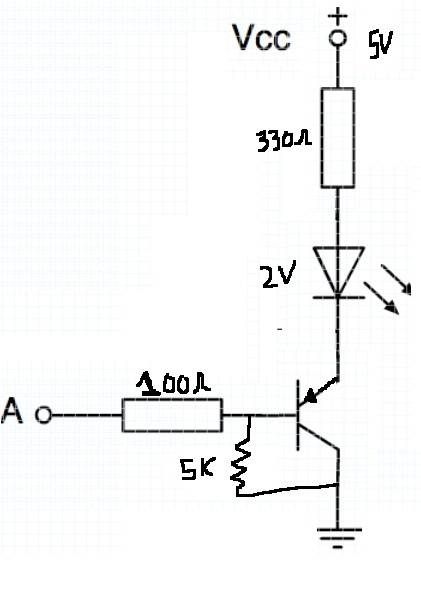# Controlling LED with Transistor

Staff Emeritus
Gold Member
I want to implement the following very simple logic:

Voltage at point "A" is high ==> LED is OFF

Voltage at point "A" is low or floating ==> LED is ON

and I came up with the attached circuit to implement it. I'm just wondering if there is anything "bad" about doing it this way, or if this circuit is OK.

#### Attachments

•led_npn.png
5.4 KB · Views: 453

MATLABdude
You should be okay with this basic inverter, assuming:
1) The LED forward voltage is higher than the BJT's saturation voltage $V_{CE(SAT)}$, and lower than $V_{CC}$, and
2) The base resistor is selected such that the BJT actually saturates

Staff Emeritus
Gold Member
You should be okay with this basic inverter, assuming:
1) The LED forward voltage is higher than the BJT's saturation voltage $V_{CE(SAT)}$, and lower than $V_{CC}$,

Okay, well I have measured a fairly large forward voltage for this LED, ~ 1.8 V, whereas it appears that the collector is pulled down to 0.2 V when conducting (I assume that this is the saturation voltage). My Vcc is 6.0 V, since I'm using 4 AA batteries.

and
2) The base resistor is selected such that the BJT actually saturates

My understanding of what saturation is is that the base current is large enough that the expected collector current IC = βIB, is so large that it cannot possibly be achieved by a voltage drop across the collector resistor. The required voltage drop would mean pulling the collector below ground, which the transistor cannot do. Instead the collector just gets pulled down to some small voltage value that is almost 0 -- the saturation voltage. It seems like with a choice of base resistor of 1k or 10k, saturation is happening easily. Am I understanding things correctly?

MATLABdude
Things sound like they're all right. When I learnt of saturation, it was "starving the collector", but it could just as easily be "stuffing the base", depending on your context and what you have available. When you design for saturation, you get to choose the value for beta, such that $\beta_{SAT} < \beta_{\circ}$